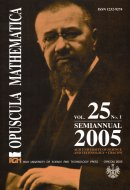Opuscula Math. 25, no. 1 (2005), 109-130

Opuscula Mathematica

# Numerical approximations of difference functional equations and applications

Zdzisław Kamont

Abstract. We give a theorem on the error estimate of approximate solutions for difference functional equations of the Volterra type. We apply this general result in the investigation of the stability of difference schemes generated by nonlinear first order partial differential functional equations and by parabolic problems. We show that all known results on difference methods for initial or initial boundary value problems can be obtained as particular cases of this general and simple result. We assume that the right hand sides of equations satisfy nonlinear estimates of the Perron type with respect to functional variables.

Keywords: functional differential equations, stability and convergence, interpolating operators, nonlinear estimates of the Perron type.

Mathematics Subject Classification: 35R10, 65M12.

Full text (pdf)

• Zdzisław Kamont
• University of Gdańsk, Institute of Mathematics, Wit Stwosz Street 57, 80-952 Gdańsk, Poland